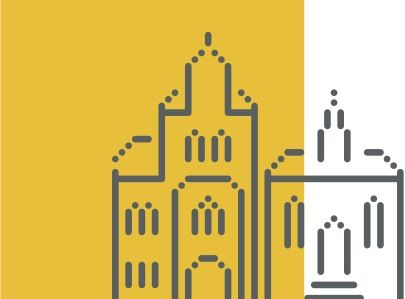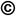TOP
##### Search the Dagstuhl Website
Looking for information on the websites of the individual seminars? - Then please:
Not found what you are looking for? - Some of our services have separate websites, each with its own search option. Please check the following list:##### Seminars
###### External resources:
• DOOR (for registering your stay at Dagstuhl)
• DOSA (for proposing future Dagstuhl Seminars or Dagstuhl Perspectives Workshops)
##### dblp
###### External resources:
• the dblp Computer Science Bibliography

### Structure and Hardness in P

##### ( Nov 06 – Nov 11, 2016 )

(Click in the middle of the image to enlarge)

##### Organizers
• (Bar-Ilan University - Ramat Gan, IL)
• (University of Michigan - Ann Arbor, US)
• (Stanford University, US)

##### Motivation

The complexity class P (polynomial time) contains a vast variety of problems of practical interest and yet relatively little is known about the structure of P, or of the complexity of many individual problems in P. It is known that there exist contrived problems requiring Ω(n1.5) time or Ω(n2) time, and yet to date no unconditional nonlinear lower bounds have been proved for any problem of practical interest. However, the last few years have seen a new resurgence in conditional lower bounds, whose validity rests on the conjectured hardness of some archetypal computational problem. This work has imbued the class P with new structure and has valuable explanatory power.

To cite a small fraction of recent discoveries, it is now known that classic dynamic programming problems such as Edit Distance, LCS, and Fréchet distance require quadratic time (based on the conjectured hardness of k-CNF-SAT), that the best known triangle enumeration algorithms are optimal (based on the hardness of 3SUM), that Valiant's context-free grammar parser is optimal (based on the hardness of k-CLIQUE), and that the best known approximate Nash equilibrium algorithm is optimal (based on the hardness of 3SAT).

This Dagstuhl Seminar will bring together top researchers in diverse areas of theoretical computer science and include a mixture of both experts and non-experts in conditional lower bounds. Some specific goals of this seminar are listed below.

• Numerous important problems (such as Linear Programming) seem insoluble in linear time, and yet no conditional lower bounds are known to explain this fact. A goal is to discover conditional lower bounds for key problems for which little is currently known.
• Recent work has been based on both traditional hardness assumptions (such as the ETH, SETH, 3SUM, and APSP conjectures) and a variety of newly considered hardness assumptions (such as the OMv conjecture, the k-CLIQUE conjecture, and the Hitting Set conjecture). Almost nothing is known about the relative plausibility of these conjectures, or if multiple conjectures are, in fact, equivalent. A goal is to discover formal relationships between the traditional and newer hardness assumptions.
• A key goal of the seminar is to disseminate the techniques used to prove conditional lower bounds, particularly to researchers from areas of theoretical computer science that have yet to benefit from this theory. To this end the seminar will include a number of tutorials from top experts in the field.

##### Summary

The complexity class P (polynomial time) contains a vast variety of problems of practical interest and yet relatively little is known about the structure of P, or of the complexity of many individual problems in P. It is known that there exist contrived problems requiring Omega(n1.5) time or Omega(n2) time, and yet to date no unconditional nonlinear lower bounds have been proved for any problem of practical interest. However, the last few years have seen a new resurgence in conditional lower bounds, whose validity rests on the conjectured hardness of some archetypal computational problem. This work has imbued the class P with new structure and has valuable explanatory power.

To cite a small fraction of recent discoveries, it is now known that classic dynamic programming problems such as Edit Distance, LCS, and Fréchet distance require quadratic time (based on the conjectured hardness of k-CNF-SAT), that the best known triangle enumeration algorithms are optimal (based on the hardness of 3-SUM), that Valiant's context-free grammar parser is optimal (based on the hardness of k-CLIQUE), and that the best known approximate Nash equilibrium algorithm is optimal (based on the hardness of 3-SAT).

This Dagstuhl Seminar will bring together top researchers in diverse areas of theoretical computer science and include a mixture of both experts and non-experts in conditional lower bounds. Some specific goals of this seminar are listed below.

• Numerous important problems (such as Linear Programming) seem insoluble in linear time, and yet no conditional lower bounds are known to explain this fact. A goal is to discover conditional lower bounds for key problems for which little is currently known.
• Recent work has been based on both traditional hardness assumptions (such as the ETH, SETH, 3SUM, and APSP conjectures) and a variety of newly considered hardness assumptions (such as the OMv conjecture, the k-CLIQUE conjecture, and the Hitting Set conjecture). Almost nothing is known about the relative plausibility of these conjectures, or if multiple conjectures are, in fact, equivalent. A goal is to discover formal relationships between the traditional and newer hardness assumptions.
• A key goal of the seminar is to disseminate the techniques used to prove conditional lower bounds, particularly to researchers from areas of theoretical computer science that have yet to benefit from this theory. To this end the seminar will include a number of tutorials from top experts in the field.Moshe Lewenstein, Seth Pettie, and Virginia Vassilevska Williams

##### Participants
• Amir Abboud (Stanford University, US) [dblp]
• Alexandr Andoni (Columbia University - New York, US) [dblp]
• Arturs Backurs (MIT - Cambridge, US) [dblp]
• Andreas Björklund (Lund University, SE) [dblp]
• Karl Bringmann (MPI für Informatik - Saarbrücken, DE) [dblp]
• Maike Buchin (Ruhr-Universität Bochum, DE) [dblp]
• Yi-Jun Chang (University of Michigan - Ann Arbor, US) [dblp]
• Raphaël Clifford (University of Bristol, GB) [dblp]
• Marek Cygan (University of Warsaw, PL) [dblp]
• Søren Dahlgaard (University of Copenhagen, DK) [dblp]
• Holger Dell (Universität des Saarlandes, DE) [dblp]
• Fedor V. Fomin (University of Bergen, NO) [dblp]
• Sebastian Forster (MPI für Informatik - Saarbrücken, DE) [dblp]
• Omer Gold (Tel Aviv University, IL) [dblp]
• Isaac Goldstein (Bar-Ilan University - Ramat Gan, IL) [dblp]
• Thore Husfeldt (IT University of Copenhagen, DK) [dblp]
• Mathias Bæk Tejs Knudsen (University of Copenhagen, DK) [dblp]
• Young Kun Ko (Princeton University, US) [dblp]
• Tsvi Kopelowitz (University of Michigan - Ann Arbor, US) [dblp]
• Robert Krauthgamer (Weizmann Institute - Rehovot, IL) [dblp]
• Marvin Künnemann (University of California - San Diego, US) [dblp]
• Moshe Lewenstein (Bar-Ilan University - Ramat Gan, IL) [dblp]
• Andrea Lincoln (Stanford University, US) [dblp]
• Veronika Loitzenbauer (Universität Wien, AT) [dblp]
• Aleksander Madry (MIT - Cambridge, US) [dblp]
• Kurt Mehlhorn (MPI für Informatik - Saarbrücken, DE) [dblp]
• Ivan Mikhailin (University of California - San Diego, US) [dblp]
• Danupon Nanongkai (KTH Royal Institute of Technology - Stockholm, SE) [dblp]
• Ramamohan Paturi (University of California - San Diego, US) [dblp]
• Seth Pettie (University of Michigan - Ann Arbor, US) [dblp]
• Ely Porat (Bar-Ilan University - Ramat Gan, IL) [dblp]
• Vijaya Ramachandran (University of Texas - Austin, US) [dblp]
• Liam Roditty (Bar-Ilan University - Ramat Gan, IL) [dblp]
• Aviad Rubinstein (University of California - Berkeley, US) [dblp]
• Thatchaphol Saranurak (KTH Royal Institute of Technology - Stockholm, SE) [dblp]
• Stefan Schneider (University of California - San Diego, US) [dblp]
• Arkadiusz Socala (University of Warsaw, PL) [dblp]
• Virginia Vassilevska Williams (Stanford University, US) [dblp]
• Joshua R. Wang (Stanford University, US) [dblp]
• Oren Weimann (University of Haifa, IL) [dblp]
• Philip Wellnitz (MPI für Informatik - Saarbrücken, DE) [dblp]
• Huacheng Yu (Stanford University, US) [dblp]

##### Classification
• data structures / algorithms / complexity

##### Keywords
• classifying P
• lower bounds
• hardness assumptions
• algorithmic equivalences